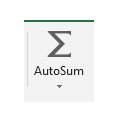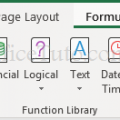## Excel Sum function

In this tutorial, we will see different methods of how to sum in Excel using the AutoSum tool and the SUM function.## Excel formulas and functions – All you want to know about them

In this tutorial, we will talk about Excel formulas and functions. Precisely, how to create or insert a formula, how to edit it, how to use functions in a formula, how to copy a formula down a column or along a row, and how to show formulas in Excel.